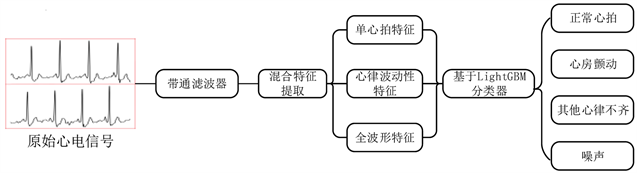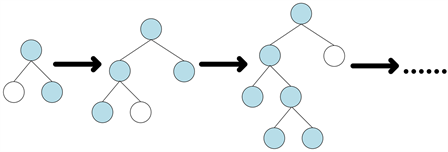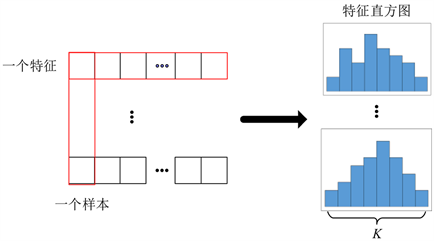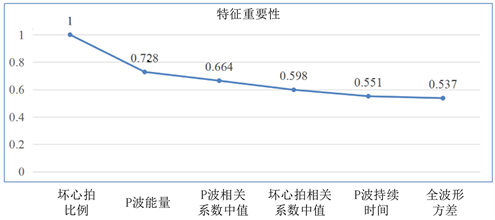# 基于LightGBM的心电信号分类算法ECG Signal’s Classification Algorithm Based on LightGBM

DOI: 10.12677/JISP.2020.93020, PDF, HTML, XML, 下载: 80  浏览: 131

Abstract: Electrocardiogram (ECG) has been widely used in the diagnosis of arrhythmia such as sinus tachycardia, ventricular premature beats and atrial fibrillation, and has shown great clinical application value in the diagnosis and analysis of heart diseases. In order to improve the classification performance of ECG computer-aided diagnosis, an ECG signal classification algorithm based on LightGBM was proposed. The algorithm extracts single heart beat features, heart rhythm volatility features and full waveform features from ECGs to establish a mixed feature set, and uses LightGBM to classify four categories: normal heart beat, atrial fibrillation, other arrhythmia, and noise. Finally, the performance parameter  of the algorithm reached 0.824 on PhysioNet/CinC Challenge dataset, which was better than CART and CatBoost. At the same time, in order to accelerate the speed of ECG feature extraction, this paper screens the key features according to the importance of features to reduce the number of features required for classification, and the feature extraction time is reduced to 17.8% while maintaining the performance of classification.

1. 引言

2. 算法框架Figure 1. The flow of ECG signal’s classification algorithm based on LightGBM

3. 算法介绍

3.1. LightGBM算法

LightGBM是由微软提供的针对梯度提升决策树(Gradient Boosting Decision Tree, GBDT)的改进算法，具有内存使用低、训练速度快和准确率高等优点 。LightGBM算法抛弃了传统GBDT算法按层生长(level-wise)的决策树生长策略，采用带有深度限制的按叶子生长(leaf-wise)策略。Leaf-wise策略分裂叶子节点的过程如图2所示，每次从当前所有叶子中找到分裂增益最大的一个叶子，然后分裂，如此循环。这样做可以在相同分裂次数情况下得到误差更低，性能更好的决策树，但是也容易长出深度较深的决策树因其过拟合，所以会增加一个决策树深度限制来防治过拟合的出现。Figure 2. The leaf nodes’ splitting process of leaf-wise strategyFigure 3. The process of feature histogram

GOSS算法的改进在于仅使用部分训练样本来估算整个训练样本的分裂增益，从而提升LightGBM的训练速度。假设存在一个训练样本集 $S=\left\{{x}_{1},{x}_{2},\cdots ,{x}_{n}\right\}$，特征维度为 。每次梯度迭代中，训练样本集 $S$ 对模型输出的负向梯度记为 $\left\{{g}_{1},{g}_{2},\cdots ,{g}_{n}\right\}$。GOSS算法会先对训练样本集 $S$ 的梯度绝对值进行降序排列，然后选取前 $a×100%$ 梯度绝对值较大的样本作为一个新的子集 $A$ ；剩余的后 $\left(1-a\right)×100%$ 梯度绝对值较小的样本集合记为 ${A}^{c}$，并从中随机采样 $b×|{A}^{c}|$ 个样本作为子集 $B$ ；最后使用样本集合 $A\cup B$ 来计算信息增益估计值 ${\stackrel{˜}{V}}_{j}\left(d\right)$，并根据该增益值来进行分割点的选择。 ${\stackrel{˜}{V}}_{j}\left(d\right)$ 的定义如公式(1)所示：

${\stackrel{˜}{V}}_{j}\left(d\right)=\frac{1}{n}\left(\frac{{\left({\sum }_{{x}_{i}\in {A}_{l}}{g}_{i}+\frac{1-a}{b}{\sum }_{{x}_{i}\in {B}_{l}}{g}_{i}\right)}^{2}}{{n}_{l}^{j}\left(d\right)}+\frac{{\left({\sum }_{{x}_{i}\in {A}_{r}}{g}_{i}+\frac{1-a}{b}{\sum }_{{x}_{i}\in {B}_{r}}{g}_{i}\right)}^{2}}{{n}_{l}^{j}\left(d\right)}\right)$ (1)

3.2. 混合特征提取

4. 性能试验

4.1. 实验数据集Table 1. The distribution information 2017 PhysioNet/CinC Challenge dataset training set

4.2. 评价指标

$\frac{1}{{F}_{1}}=\frac{1}{2}\cdot \left(\frac{1}{P}+\frac{1}{R}\right)$ (2)

${F}_{1n}=\frac{2×{N}_{n}}{\sum N+\sum n}$ (3)

${F}_{1a}=\frac{2×{A}_{a}}{\sum A+\sum a}$ (4)

${F}_{1o}=\frac{2×{O}_{o}}{\sum O+\sum o}$ (5)

${F}_{1p}=\frac{2×{P}_{p}}{\sum P+\sum p}$ (6)

$F{1}_{nao}=\frac{{F}_{1n}+{F}_{1a}+{F}_{1o}}{3}$ (7)

4.3. 算法分类精度实验Table 2. The comparison of model performance’s cross validation results

4.4. 算法分类速度提升实验Figure 4. The first six features of mixed features and their importanceTable 3. The performance comparison before and after feature selection

5. 结论

NOTES

*通讯作者。

  Rajpurkar, P., Hannun, A.Y., Haghpanahi, M., et al. (2017) Cardiologist-Level Arrhythmia Detection with Convolutional Neural Networks.  卢喜烈. 现代心电图诊断大全[M]. 北京: 科学技术文献出版社, 1997.  Tateno, K. and Glass, L. (2001) Automatic Detection of Atrial Fibrillation Using the Coefficient of Variation and Density Histograms of RR and deltaRR Intervals. Medical & Biological Engineering & Computing, 39, 664-671. https://doi.org/10.1007/BF02345439  宋莉, 孟庆建, 张光玉, 等. 基于波形特征和SVM的心电信号自动分类方法研究[J]. 中国医学物理学杂志, 2010(4): 2043-2046.  Elhaj, F.A., Salim, N., Harris, A.R., et al. (2016) Arrhythmia Recognition and Classification Using Combined Linear and Nonlinear Features of ECG Signals. Computer Methods & Programs in Biomedicine, 127, 52-63. https://doi.org/10.1016/j.cmpb.2015.12.024  颜昊霖, 安勇, 王宏飞, 等. 基于卷积神经网络的心电特征提取[J]. 计算机工程与设计, 2017(4): 1024-1028.  Kiranyaz, S., Ince, T. and Gabbouj, M. (2016) Real-Time Patient-Specific ECG Classification by 1D Convolutional Neural Networks. IEEE Transactions on Biomedical Engineering, 63, 664-675. https://doi.org/10.1109/TBME.2015.2468589  王润. 心电信号的预处理算法分析[J]. 现代计算机(专业版), 2018(7): 33-36.  Barhatte, A.S., Ghongade, R. and Tekale, S.V. (2016) Noise Analysis of ECG Signal Using Fast ICA. 2016 Conference on Advances in Signal Processing (CASP) Cummins College of Engineering for Women, Pune, 9-11 Jun 2016, 1-5. https://doi.org/10.1109/CASP.2016.7746149  Ke, G., Meng, Q., Finley, T.W., et al. (2017) LightGBM: A Highly Efficient Gradient Boosting Decision Tree. Neural Information Processing Systems, Long Beach, CA, 4-9 December 2017, 3149-3157.  Zhou, Z.-H. (2012) Ensemble Methods: Foundations and Algorithms. CRC Press, New York, 99-118. https://doi.org/10.1201/b12207